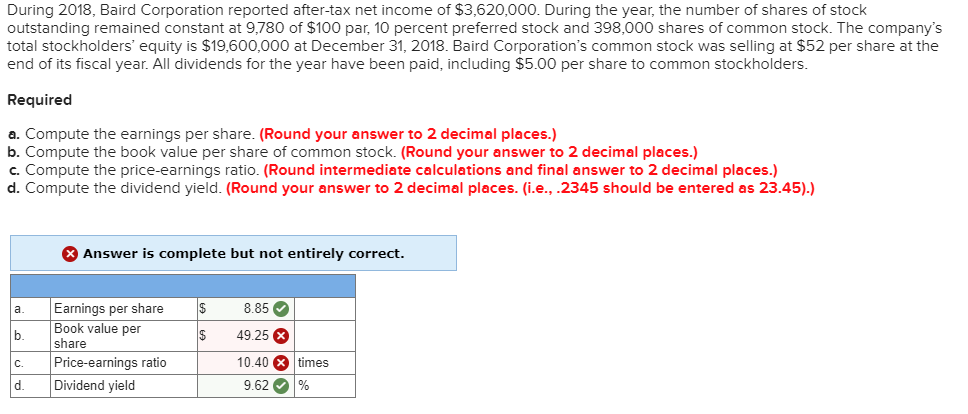# During 2018, Baird Corporation reported after-tax net income of $3,620,000. During the year, the number of shares of stock outstanding remained constant at 9,780 of$100 par, 10 percent preferred stock and 398,000 shares of common stock. The company's total stockholders' equity is $19,600,000 at December 31, 2018. Baird Corporation's common stock was selling at$52 per share at the end of its fiscal year. All dividends for the year have been paid, including $5.00 per share to common stockholders. Required a. Compute the earnings per share. (Round your answer to 2 decimal places.) b. Compute the book value per share of common stock. (Round your answer to 2 decimal places.) c. Compute the price-earnings ratio. (Round intermediate calculations and final answer to 2 decimal places.) d. Compute the dividend yield. (Round your answer to 2 decimal places. (i.e., .2345 should be entered as 23.45).) Answer is complete but not entirely correct. Earnings per share Book value per share Price-earnings ratio Dividend yield 8.85 а. b. 49.25 10.40 times C. d. 9.62 Question During 2018, Baird Corporation reported after-tax net income of$3,620,000. During the year, the number of shares of stock outstanding remained constant at 9,780 of $100 par, 10 percent preferred stock and 398,000 shares of common stock. The company’s total stockholders’ equity is$19,600,000 at December 31, 2018. Baird Corporation’s common stock was selling at $52 per share at the end of its fiscal year. All dividends for the year have been paid, including$5.00 per share to common stockholders.

Required

Compute the earnings per share. (Round your answer to 2 decimal places.)

Compute the book value per share of common stock. (Round your answer to 2 decimal places.)

Compute the price-earnings ratio. (Round intermediate calculations and final answer to 2 decimal places.)

Compute the dividend yield. (Round your answer to 2 decimal places. (i.e., .2345 should be entered as 23.45).)help_outlineImage TranscriptioncloseDuring 2018, Baird Corporation reported after-tax net income of $3,620,000. During the year, the number of shares of stock outstanding remained constant at 9,780 of$100 par, 10 percent preferred stock and 398,000 shares of common stock. The company's total stockholders' equity is $19,600,000 at December 31, 2018. Baird Corporation's common stock was selling at$52 per share at the end of its fiscal year. All dividends for the year have been paid, including \$5.00 per share to common stockholders. Required a. Compute the earnings per share. (Round your answer to 2 decimal places.) b. Compute the book value per share of common stock. (Round your answer to 2 decimal places.) c. Compute the price-earnings ratio. (Round intermediate calculations and final answer to 2 decimal places.) d. Compute the dividend yield. (Round your answer to 2 decimal places. (i.e., .2345 should be entered as 23.45).) Answer is complete but not entirely correct. Earnings per share Book value per share Price-earnings ratio Dividend yield 8.85 а. b. 49.25 10.40 times C. d. 9.62 fullscreen

1 Rating

### Want to see this answer and more?

Experts are waiting 24/7 to provide step-by-step solutions in as fast as 30 minutes!*